# GSEB Solutions Class 12 Maths Chapter 7 Integrals Ex 7.5

Gujarat Board GSEB Textbook Solutions Class 12 Maths Chapter 7 Integrals Ex 7.5 Textbook Questions and Answers.

## Gujarat Board Textbook Solutions Class 11 Maths Chapter 7 Integrals Ex 7.5

Integrate the following functions:
Question 1.
$$\frac{x}{(x+1)(x+2)}$$
Solution:
$$\frac{x}{(x+1)(x+2)}$$ may be written as
$$\frac{x}{(x+1)(x+2)}$$ = $$\frac{A}{x+1}$$ + $$\frac{B}{x+2}$$
∴ x = A(x + 2) + B(x + 1)
Put x = – 1, – 1 = A(- 1 + 2) + 0 = A ∴ A = – 1.
Put x = – 2, – 2 = 0 + B(- 2 + 1) = – B ∴ B = 2.
∴ $$\frac{x}{(x+1)(x+2)}$$ = – $$\frac{1}{x+1}$$ + $$\frac{2}{x+2}$$.
Integrating both sides, we get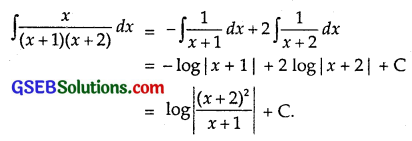Question 2.
$$\frac{1}{x^{2}-9}$$
Solution:
Let $$\frac{1}{x^{2}-9}$$ = $$\frac{1}{(x-3)(x+3)}$$ = $$\frac{A}{x-3}$$ + $$\frac{B}{x+3}$$.
⇒ 1 = A(x + 3) + B(x – 3) ……………… (1)
Putting x = 3 in (1), we get:
1 = A(3 + 3) ⇒ A = $$\frac{1}{6}$$.
Putting x = – 3 in (1), we get:
1 = B(- 3 – 3) ⇒ B = – $$\frac{1}{6}$$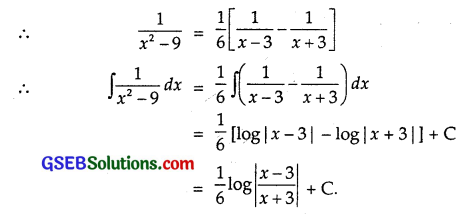Question 3.
$$\frac{3x-1}{(x-1)(x-2)(x-3)}$$
Solution:
Let $$\frac{3x-1}{(x-1)(x-2)(x-3)}$$ = $$\frac{A}{x-1}$$ + $$\frac{B}{x-2}$$ + $$\frac{C}{x-3}$$.
⇒ 3x – 1 = A(x – 2)(x – 3) + B(x – 1)(x – 3) + C(x – 1)(x – 2) ……………….. (1)
Putting x = 1 in (1) we get:
3 – 1 = A(1 – 2)(1 – 3)
⇒ 2 = A(- 1)(- 2) ⇒ A = 1.
Putting x = 2 in (1), we get:
6 – 1 = B(2 – 1)(2 – 3)
⇒ 5 = B(1)(- 1) ⇒ B = – 5.
Putting x = 3 in (1), we get:
9 – 1 = C(3 – 1)(3 – 2)
⇒ 8 = C(2)(1) ⇒ C = 4.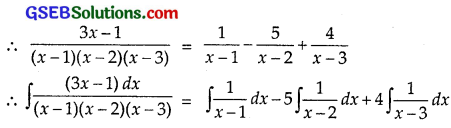= log |x – 1| – 5 log |x – 2| + 4 log |x – 3| + C.Question 4.
$$\frac{x}{(x-1)(x-2)(x-3)}$$
Solution:
Let $$\frac{x}{(x-1)(x-2)(x-3)}$$ = $$\frac{A}{x-1}$$ + $$\frac{B}{x-2}$$ + $$\frac{C}{x-3}$$.
⇒ x = A(x – 2)(x – 3) + B(x – 1)(x – 3) + C(x – 1)(x – 2) …………. (1)
Putting x = 1 in (1), we get:
1 = A(1 – 2)(1 – 3)
⇒ A = $$\frac{1}{2}$$
Putting x = 2 in (1), we get:
2 = B(2 – 1)(2 – 3) ⇒ B = – 2
Putting x = 3 in (1), we get:
3 = C(3 – 1)(3 – 2) ⇒ C = $$\frac{3}{2}$$.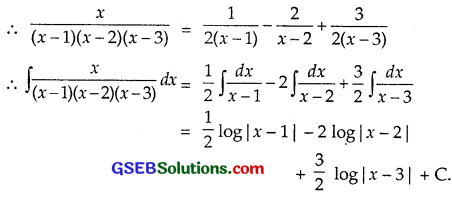Question 5.
$$\frac{2 x}{x^{2}+3 x+2}$$
Solution: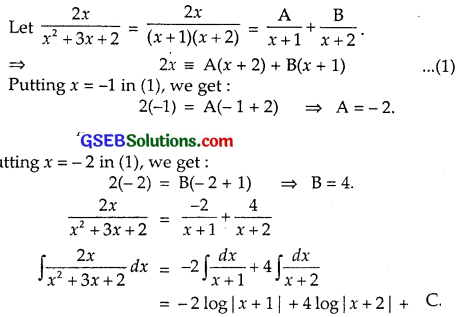Question 6.
$$\frac{1-x^{2}}{x(1-2 x)}$$
Solution:
Since $$\frac{1-x^{2}}{x(1-2 x)}$$ = $$\frac{1-x^{2}}{x-2 x^{2}}$$ is an improper function,
therefore we convert it into a proper function. Divide 1 – x2 by x – 2x2 by long division method, we get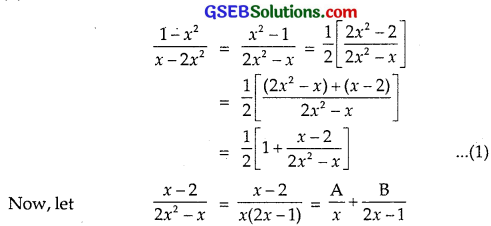⇒ x – 2 = A(2x – 1) + Bx ………………. (2)
Puttting x = 0 in (2), we get:
– 2 = A(- 1) ⇒ A = 2.
Putting x = $$\frac{1}{2}$$ in (2), we get: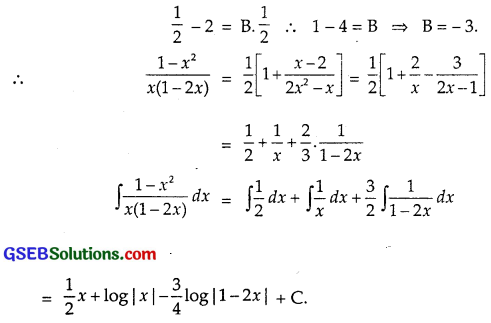Question 7.
$$\frac{x}{\left(x^{2}+1\right)(x-1)}$$
Solution:
$$\frac{x}{\left(x^{2}+1\right)(x-1)}$$ may be written as
$$\frac{x}{\left(x^{2}+1\right)(x-1)}$$ = $$\frac{A}{x-1}$$ + $$\frac{B x+C}{x^{2}-1}$$.
∴ x = A(x2 + 1) + (Bx + C)(x – 1)
= A(x2 + 1) + B(x2 – x) + C(x – 1)
Put x = 1, 1 = A(1 + 1) ⇒ 1 = 2A or A = $$\frac{1}{2}$$
Comparing the coefficients of x2, we get
0 = A + B
∴ B = – A = – $$\frac{1}{2}$$.
Comparing the constants, we get
0 = A – C ∴ C = A = $$\frac{1}{2}$$.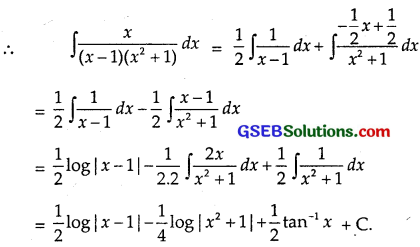Question 8.
$$\frac{x}{(x-1)^{2}(x+2)}$$
Solution:
Let $$\frac{x}{(x-1)^{2}(x+2)}$$ = $$\frac{A}{x-1}$$ + $$\frac{B}{(x-1)^{2}}$$ + $$\frac{C}{x+2}$$.
⇒ x = A(x – 1)(x + 2) + B(x + 2) + C(x – 1)2 …………….. (1)
Putting x = 1 in (1), we get:
1 = B(1 + 2) ⇒ B = $$\frac{1}{3}$$
Putting x = – 2 in (1), we get:
– 2 = C(- 2 – 1)2 ⇒ C = – $$\frac{-2}{9}$$
Comparing co-efficients of x2 on both sides of (1), we have:
0 = A + C ⇒ A – C = $$\frac{2}{9}$$.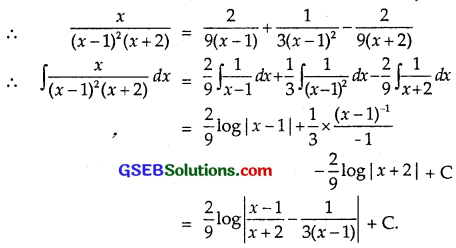Question 9.
$$\frac{3 x+5}{x^{3}-x^{2}-x+1}$$
Solution: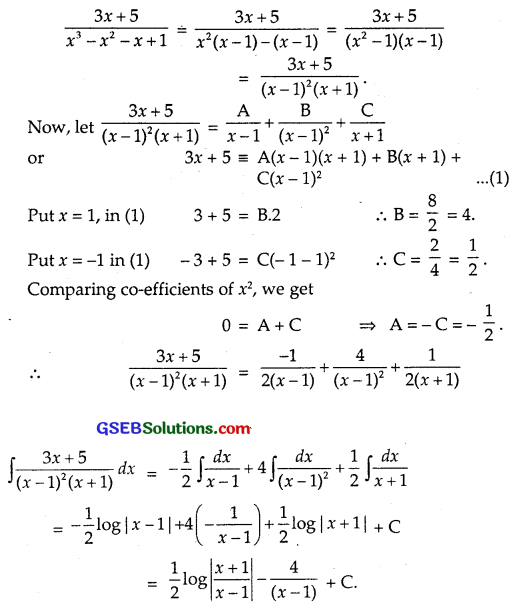Question 10.
$$\frac{2 x-3}{\left(x^{2}-1\right)(2 x+3)}$$
Solution:
Let $$\frac{2 x-3}{\left(x^{2}-1\right)(2 x+3)}$$ = $$\frac{2x-3}{(x-1)(x+1)(2x+3)}$$
= $$\frac{A}{x-1}$$ + $$\frac{B}{x+1}$$ + $$\frac{C}{2x+3}$$
⇒ 2x – 3 = A(x + 1)(2x + 3) + B(x – 1)(2x + 3) + C(x – 1)(x + 1) …………….. (1)
Putting x = 1 in (1), we get
2(1) – 3 = A(1 + 1)(2 + 3)
⇒ – 1 = A(2)(5) ⇒ A = – $$\frac{1}{10}$$.
Putting x = – 1 in (1), we get
– 2 – 3 = B(- 1 – 1)(- 2 + 3)
⇒ – 5 = B(- 2)(1) ⇒ B = $$\frac{5}{2}$$
Putting x = – $$\frac{3}{2}$$ in (1), we get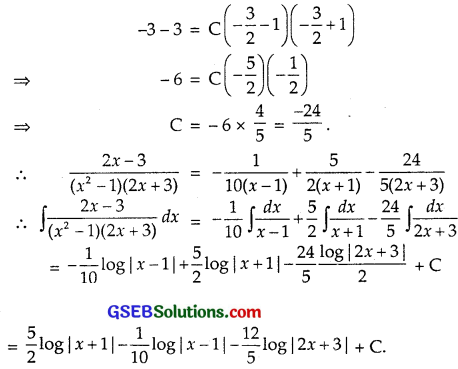Question 11.
$$\frac{5 x}{(x+1)\left(x^{2}-4\right)}$$
Solution:
Let $$\frac{5 x}{(x+1)\left(x^{2}-4\right)}$$ = $$\frac{5x}{(x+1)(x+2)(x-2)}$$
= $$\frac{A}{x+1}$$ + $$\frac{B}{x+2}$$ + $$\frac{C}{x-2}$$
⇒ 5x = A(x + 2)(x – 2) + B(x + 1)(x – 2) + C(x + 1)(x + 2) ………….. (1)
Putting x = – 1 in (1), we get
– 5 = A(- 1 + 2)(- 1 – 2) ⇒ A = $$\frac{5}{3}$$.
Putting x = – 2 in (1), we get
– 10 = B(- 2 + 1)(- 2 – 2) ⇒ B = – $$\frac{5}{2}$$
Putting x = 2 in (1), we get
10 = C(2 + 1)(2 + 2) ⇒ C = $$\frac{5}{6}$$.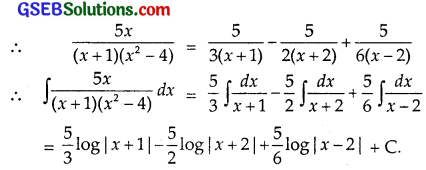Question 12.
$$\frac{x^{3}+x+1}{x^{2}-1}$$
Solution:
Since $$\frac{x^{3}+x+1}{x^{2}-1}$$ is an improper fraction, therefore we convert it into a proper fraction.
Dividing the polynomial function x3 + x + 1 by x2 – 1 by long division, we get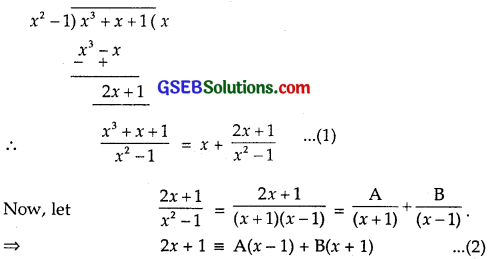Putting x = – 1 in (2), we get
⇒ 2x + 1 = A(- 1 – 1) ⇒ A = $$\frac{-1}{-2}$$ = $$\frac{1}{2}$$.
Putting x = 1 in (2), we get
2 + 1 = B(1 + 1) ⇒ B = $$\frac{3}{2}$$.
∴ $$\frac{2 x+1}{x^{2}-1}$$ = $$\frac{1}{2(x+1)}$$ + $$\frac{3}{2(x-1)}$$ …………. (3)
From (1) and (3),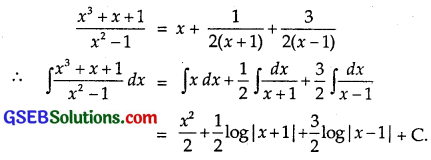Question 13.
$$\frac{2}{(1-x)\left(1+x^{2}\right)}$$
Solution:
Let $$\frac{2 x+1}{x^{2}-1}$$ = $$\frac{A}{1-x}$$ + $$\frac{B x+C}{1+x^{2}}$$.
⇒ 2 = A(1 + x2) + (Bx + C)(1 – x) …………… (1)
Putting x = 1 in (1), we get:
2 = A(1 + 1) ⇒ A = 1.
Comparing co-efficients of x2 and the constant terms on both sides, we get:
0 = A – B and 2 = A + C
⇒ B = A = 1 and 2 = 1 + C.
⇒ C = 1.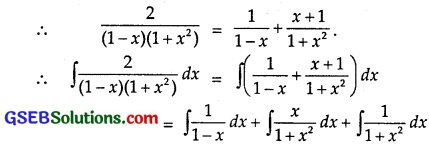Question 14.
$$\frac{3 x-1}{(x+2)^{2}}$$
Solution: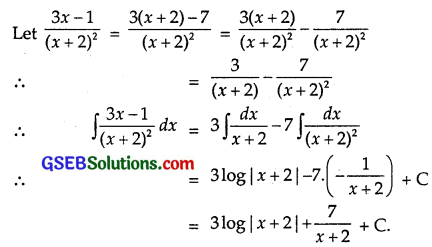Question 15.
$$\frac{1}{x^{4}-1}$$
Solution: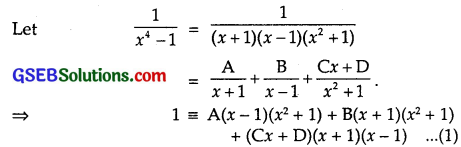Putting x = – 1 in (1) we get:
1 = A(- 1 – 1)(1 + 1)
⇒ 1 = A(- 4) ⇒ A = – $$\frac{1}{4}$$
Putting x = 1 in (1), we get:
1 = B(1 + 1)(1 + 1)
⇒ 1 = B(2)(2) ⇒ B = $$\frac{1}{4}$$.
Comparing the coefficients of x3 and constants in (1) on both sides, we get
0 = A + B + C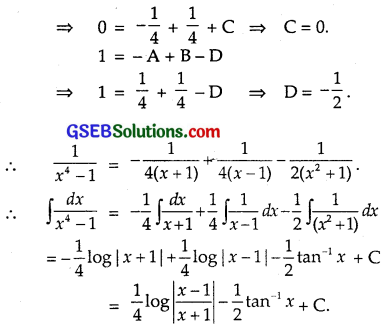Question 16.
$$\frac{1}{x\left(x^{n}+1\right)}$$
Solution:
⇒ 1 = A(t + 1) + Bt …………….. (2)
Putting t = 0 in (2), we get:
1 = B(- 1) ⇒ B = – 1
∴ (1) gives: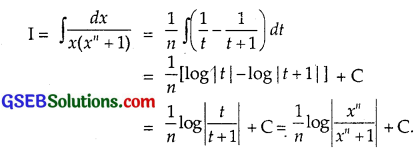Question 17.
$$\frac{cosx}{(1-sin2x)(2-sinx)}$$
Solution:
Put sin x = t so that cos x dx = dt.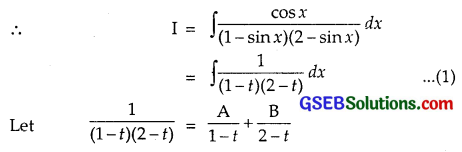⇒ 1 = A(2 – t) + B(1 – t) …………….. (2)
Putting t = 1 in (2) we get:
1 = A(2 – 1) ⇒ A = 1.
Putting t = 2 in (2), we get:
1 = B(1 – 2) ⇒ B = – 1.
∴ (1) gives: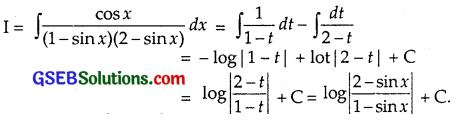Question 18.
$$\frac{\left(x^{2}+1\right)\left(x^{2}+2\right)}{\left(x^{2}+3\right)\left(x^{2}+4\right)}$$
Solution:Consider partial fractions of
$$\frac{(2y+5)}{(y+3)(y+5)}$$ = $$\frac{A}{y+3}$$ + $$\frac{B}{y+4}$$
Put y = – 3 in (2), – 6 + 5 = A.1 ∴ A = – 1.
Put y = – 4 in (2), – 8 + 5 = B(- 4 + 3)
or – 3 = – B ∴ B = 3.
Putting these values in (1), we get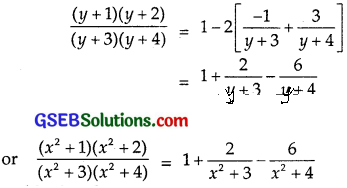Integrated both sides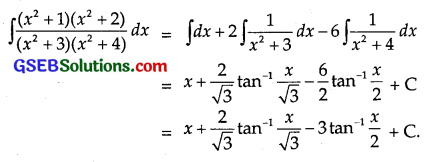Question 19.
$$\frac{2 x}{\left(x^{2}+1\right)\left(x^{2}+3\right)}$$
Solution: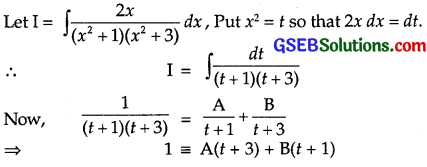Put t = – 1, 1 = A(- 1 + 3) = 2A ∴ A = $$\frac{1}{2}$$
Put t = – 3, 1 = B(- 3 + 1) = – 2B ∴ B = – $$\frac{1}{2}$$.
∴ $$\frac{1}{(t+1)(t+3)}$$ = $$\frac{1}{2}$$
Integrating both sides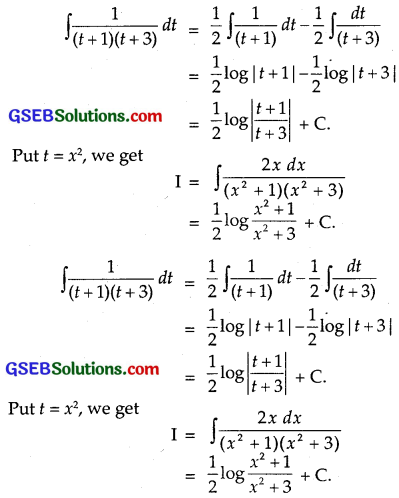Question 20.
$$\frac{1}{x\left(x^{4}-1\right)}$$
Solution: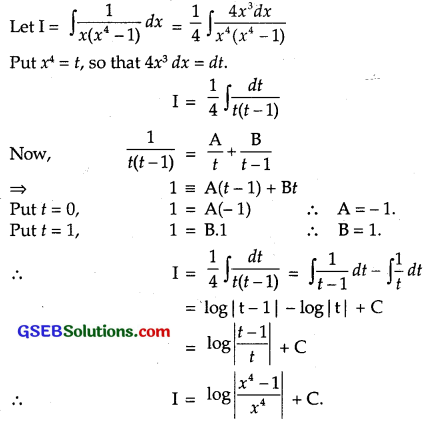Question 21.
$$\frac{1}{e^{x}-1}$$
Solution: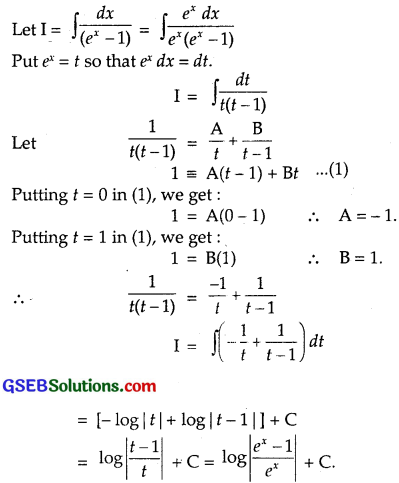Choose the correct answer in each of the following questions 22 and 23:
Question 22.
∫ $$\frac{xdx}{(x-1)(x-2)}$$ equals
(A) log |$$\frac{(x-1)^{2}}{x-2}$$| + C
(B) log |$$\frac{(x-2)^{2}}{x-1}$$| + C
(C) log |($$\frac{(x-1)^{2}}{x-2}$$| + C
(D) log |(x – 1)(x – 2)| + C
Solution:
Let $$\frac{x}{(x-1)(x-2)}$$ = $$\frac{A}{x-1}$$ + $$\frac{B}{x-2}$$
∴ x = A(x – 2) + B(x – 1)
Put x = 1, 1 = A(1 – 2) = – A ∴ A = – 1
Put x = 2, 2 = B(2 – 1) = B ∴ B = 2.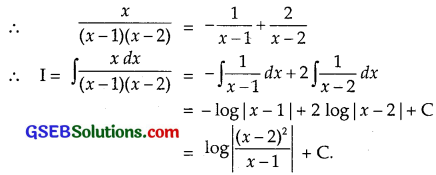∴ Part (B) is the correct answer.Question 23.
∫ $$\frac{d x}{x\left(x^{2}+1\right)}$$ equals
(A) log|x| – $$\frac{1}{2}$$ log |x2 + 1| + C
(B) log |x| + $$\frac{1}{2}$$ log|x2 + 1| + C
(C) – log |x| + $$\frac{1}{2}$$ log |x2 + 1| + C
(D) $$\frac{1}{2}$$ log|x| + log |x2 + 1| + C
Solution: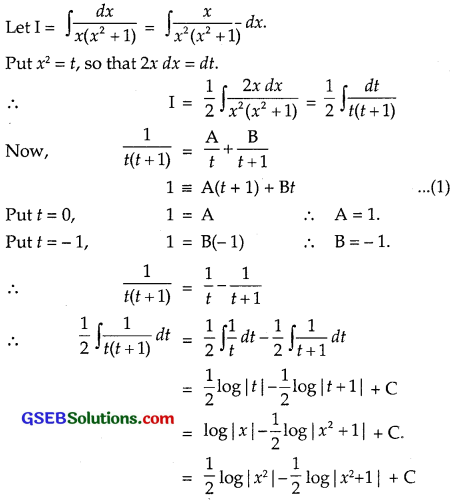∴ Part (A) is the correct answer.